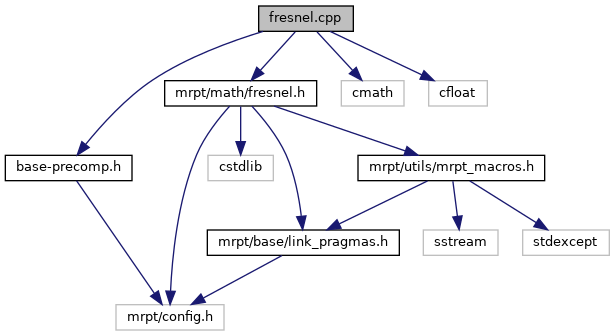Main MRPT website > C++ reference for MRPT 1.5.6
fresnel.cpp File Reference
`#include "base-precomp.h"`
`#include <mrpt/math/fresnel.h>`
`#include <cmath>`
`#include <cfloat>`
Include dependency graph for fresnel.cpp:Go to the source code of this file.

Macros

#define NUM_ASYMPTOTIC_TERMS   35

#define NUM_ASYMPTOTIC_TERMS   35

Functions

static long double Power_Series_S (long double x)

static long double xFresnel_Auxiliary_Cosine_Integral (long double x)

static long double xFresnel_Auxiliary_Sine_Integral (long double x)

static long double Power_Series_C (long double x)

static long double xChebyshev_Tn_Series (long double x, const long double a[], int degree)

long double lfresnel_sin_alt (long double x)

long double lfresnel_cos_alt (long double x)

double Fresnel_Sine_Integral (double x)

long double xFresnel_Sine_Integral (long double x)

double Fresnel_Auxiliary_Sine_Integral (double x)

static long double sin_Chebyshev_Expansion_0_1 (long double x)

static long double sin_Chebyshev_Expansion_1_3 (long double x)

static long double sin_Chebyshev_Expansion_3_5 (long double x)

static long double sin_Chebyshev_Expansion_5_7 (long double x)

static long double sin_Asymptotic_Series (long double x)

double Fresnel_Auxiliary_Cosine_Integral (double x)

static long double cos_Chebyshev_Expansion_0_1 (long double x)

static long double cos_Chebyshev_Expansion_1_3 (long double x)

static long double cos_Chebyshev_Expansion_3_5 (long double x)

static long double cos_Chebyshev_Expansion_5_7 (long double x)

static long double cos_Asymptotic_Series (long double x)

double Fresnel_Cosine_Integral (double x)

long double xFresnel_Cosine_Integral (long double x)

Variables

static long double const sqrt_2pi = 2.506628274631000502415765284811045253006L

Macro Definition Documentation

 #define NUM_ASYMPTOTIC_TERMS   35

Definition at line 1007 of file fresnel.cpp.

Referenced by cos_Asymptotic_Series(), and sin_Asymptotic_Series().

 #define NUM_ASYMPTOTIC_TERMS   35

Definition at line 1007 of file fresnel.cpp.

Function Documentation

 static long double cos_Asymptotic_Series ( long double x )
static

Definition at line 1008 of file fresnel.cpp.

References mrpt::math::factorial(), NUM_ASYMPTOTIC_TERMS, and sqrt_2pi.

Referenced by xFresnel_Auxiliary_Cosine_Integral().

 static long double cos_Chebyshev_Expansion_0_1 ( long double x )
static

Definition at line 818 of file fresnel.cpp.

References xChebyshev_Tn_Series().

Referenced by xFresnel_Auxiliary_Cosine_Integral().

 static long double cos_Chebyshev_Expansion_1_3 ( long double x )
static

Definition at line 865 of file fresnel.cpp.

References xChebyshev_Tn_Series().

Referenced by xFresnel_Auxiliary_Cosine_Integral().

 static long double cos_Chebyshev_Expansion_3_5 ( long double x )
static

Definition at line 914 of file fresnel.cpp.

References xChebyshev_Tn_Series().

Referenced by xFresnel_Auxiliary_Cosine_Integral().

 static long double cos_Chebyshev_Expansion_5_7 ( long double x )
static

Definition at line 960 of file fresnel.cpp.

References xChebyshev_Tn_Series().

Referenced by xFresnel_Auxiliary_Cosine_Integral().

 double Fresnel_Auxiliary_Cosine_Integral ( double x )

Definition at line 558 of file fresnel.cpp.

References xFresnel_Auxiliary_Cosine_Integral().

 double Fresnel_Auxiliary_Sine_Integral ( double x )

Definition at line 234 of file fresnel.cpp.

References xFresnel_Auxiliary_Sine_Integral().

 double Fresnel_Cosine_Integral ( double x )

Definition at line 633 of file fresnel.cpp.

References xFresnel_Cosine_Integral().

 double Fresnel_Sine_Integral ( double x )

Definition at line 104 of file fresnel.cpp.

References xFresnel_Sine_Integral().

 long double lfresnel_cos_alt ( long double x )

Definition at line 43 of file fresnel.cpp.

 long double lfresnel_sin_alt ( long double x )

Definition at line 29 of file fresnel.cpp.

 static long double Power_Series_C ( long double x )
static

Definition at line 702 of file fresnel.cpp.

References mrpt::math::factorial().

Referenced by lfresnel_cos_alt(), and xFresnel_Cosine_Integral().

 static long double Power_Series_S ( long double x )
static

Definition at line 172 of file fresnel.cpp.

References mrpt::math::factorial().

Referenced by lfresnel_sin_alt(), and xFresnel_Sine_Integral().

 static long double sin_Asymptotic_Series ( long double x )
static

Definition at line 498 of file fresnel.cpp.

References mrpt::math::factorial(), NUM_ASYMPTOTIC_TERMS, and sqrt_2pi.

Referenced by xFresnel_Auxiliary_Sine_Integral().

 static long double sin_Chebyshev_Expansion_0_1 ( long double x )
static

Definition at line 306 of file fresnel.cpp.

References xChebyshev_Tn_Series().

Referenced by xFresnel_Auxiliary_Sine_Integral().

 static long double sin_Chebyshev_Expansion_1_3 ( long double x )
static

Definition at line 353 of file fresnel.cpp.

References xChebyshev_Tn_Series().

Referenced by xFresnel_Auxiliary_Sine_Integral().

 static long double sin_Chebyshev_Expansion_3_5 ( long double x )
static

Definition at line 402 of file fresnel.cpp.

References xChebyshev_Tn_Series().

Referenced by xFresnel_Auxiliary_Sine_Integral().

 static long double sin_Chebyshev_Expansion_5_7 ( long double x )
static

Definition at line 449 of file fresnel.cpp.

References xChebyshev_Tn_Series().

Referenced by xFresnel_Auxiliary_Sine_Integral().

 static long double xChebyshev_Tn_Series ( long double x, const long double a[], int degree )
static

Definition at line 771 of file fresnel.cpp.

 long double xFresnel_Auxiliary_Cosine_Integral ( long double x )
static

Definition at line 593 of file fresnel.cpp.

 long double xFresnel_Auxiliary_Sine_Integral ( long double x )
static

Definition at line 271 of file fresnel.cpp.

 long double xFresnel_Cosine_Integral ( long double x )

Definition at line 661 of file fresnel.cpp.

Referenced by Fresnel_Cosine_Integral().

 long double xFresnel_Sine_Integral ( long double x )

Definition at line 132 of file fresnel.cpp.

Referenced by Fresnel_Sine_Integral().

Variable Documentation

 long double const sqrt_2pi = 2.506628274631000502415765284811045253006L
static

Definition at line 207 of file fresnel.cpp.

Referenced by cos_Asymptotic_Series(), and sin_Asymptotic_Series().

 Page generated by Doxygen 1.8.6 for MRPT 1.5.6 Git: 4c65e84 Tue Apr 24 08:18:17 2018 +0200 at mar abr 24 08:26:17 CEST 2018